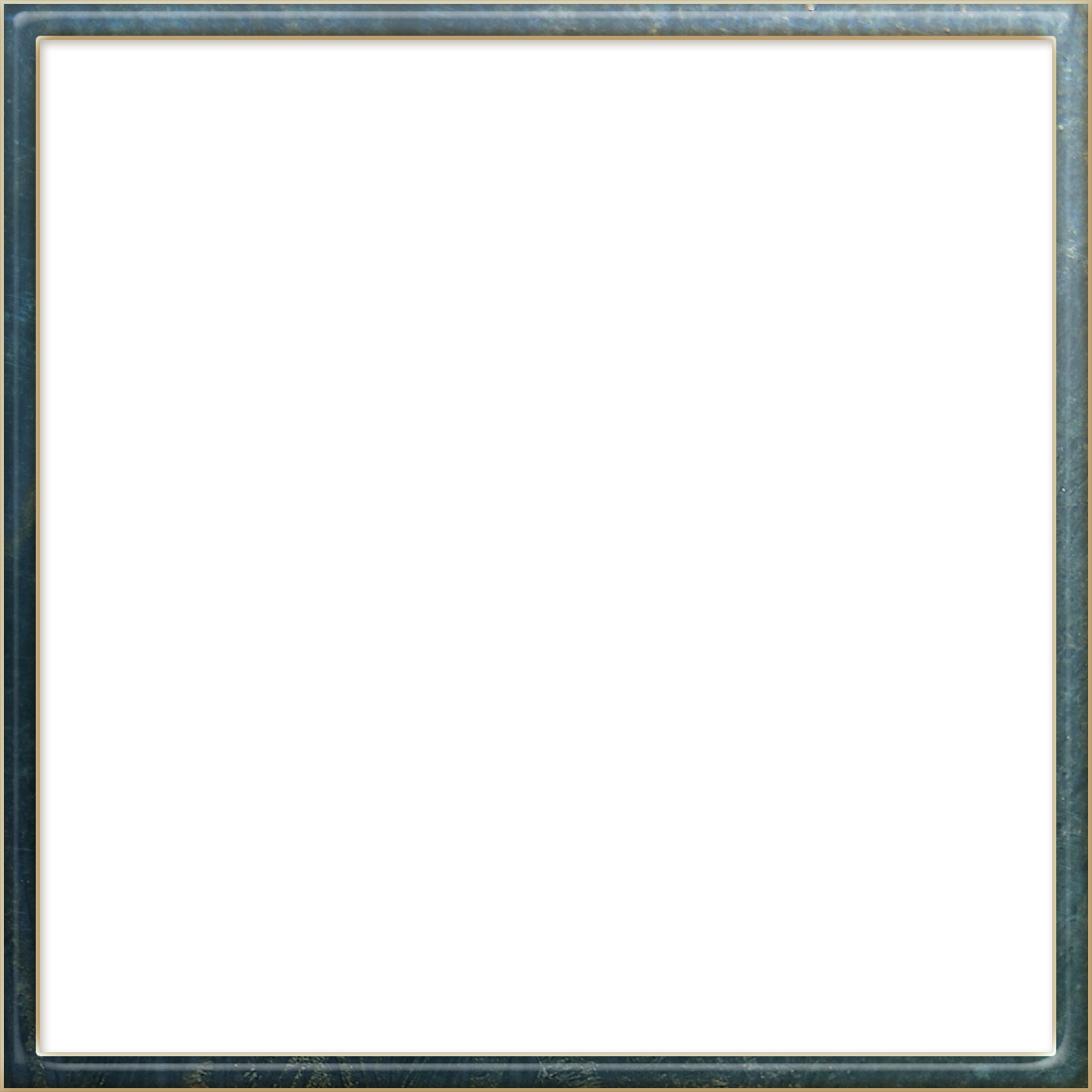Language: English | España | РусскийShare image:

Keywords: Square PNG size: 1100x1100px, Square PNG images, PNG image: Square PNG, free PNG image, Square
Image category: Square
Format: PNG image with alpha (transparent)
Resolution: 1100x1100
Size: 265 kb

# Square PNG image with transparent background | square_PNG79.png

This image has format transparent PNG with resolution 1100x1100.

# Square

In geometry, a square is a regular quadrilateral, which means that it has four equal sides and four equal angles (90-degree angles, or (100-gradian angles or right angles). It can also be defined as a rectangle in which two adjacent sides have equal length. A square with vertices ABCD would be denoted square ABCD.

A square is a special case of a rhombus (equal sides, opposite equal angles), a kite (two pairs of adjacent equal sides), a trapezoid (one pair of opposite sides parallel), a parallelogram (all opposite sides parallel), a quadrilateral or tetragon (four-sided polygon), and a rectangle (opposite sides equal, right-angles) and therefore has all the properties of all these shapes

Every acute triangle has three inscribed squares (squares in its interior such that all four of a square's vertices lie on a side of the triangle, so two of them lie on the same side and hence one side of the square coincides with part of a side of the triangle). In a right triangle two of the squares coincide and have a vertex at the triangle's right angle, so a right triangle has only two distinct inscribed squares. An obtuse triangle has only one inscribed square, with a side coinciding with part of the triangle's longest side.

The fraction of the triangle's area that is filled by the square is no more than 1/2.

In non-Euclidean geometry, squares are more generally polygons with 4 equal sides and equal angles.

In spherical geometry, a square is a polygon whose edges are great circle arcs of equal distance, which meet at equal angles. Unlike the square of plane geometry, the angles of such a square are larger than a right angle. Larger spherical squares have larger angles.

In hyperbolic geometry, squares with right angles do not exist. Rather, squares in hyperbolic geometry have angles of less than right angles. Larger hyperbolic squares have smaller angles.